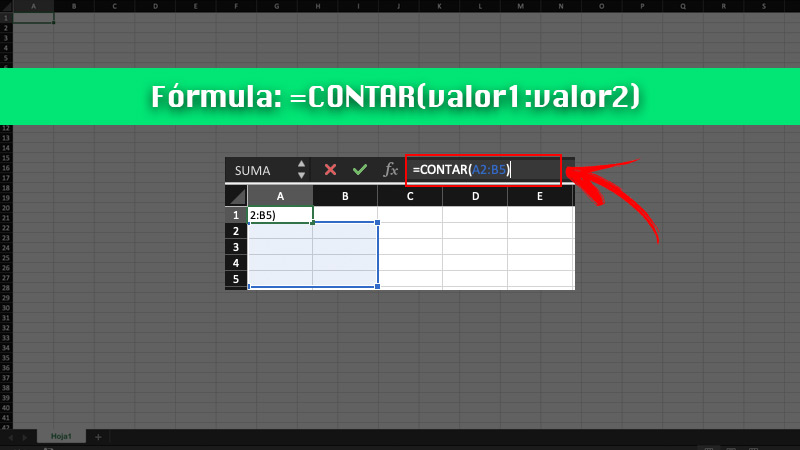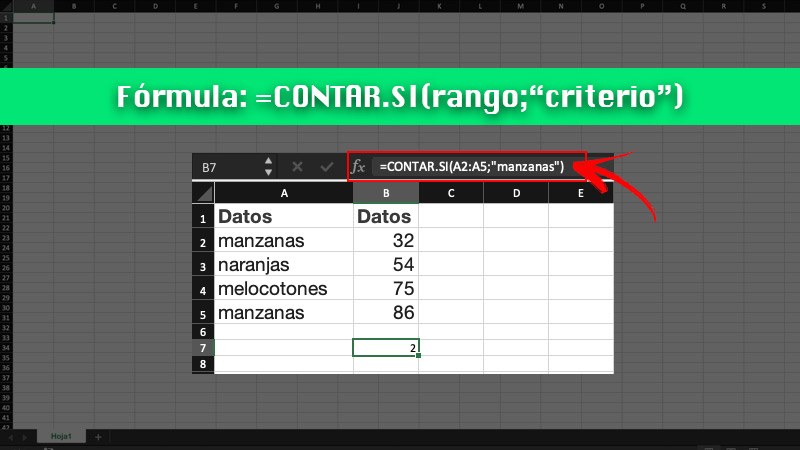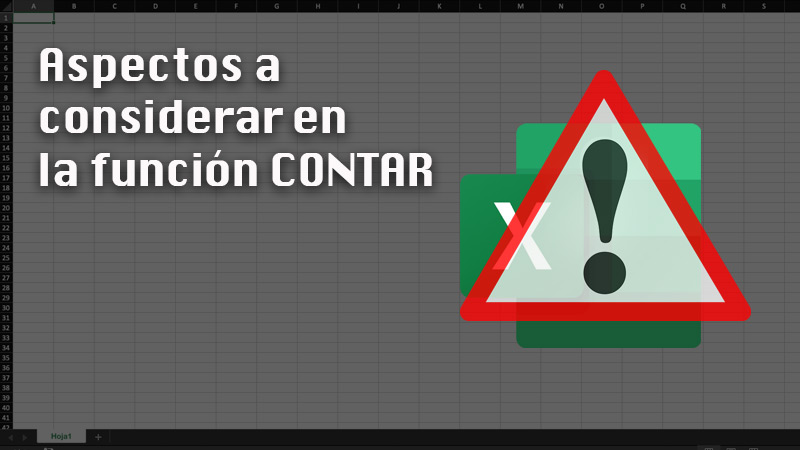COUNT function in Excel  Step by Step Guide ▷ 2020

Excel is the world’s leading spreadsheet program. It has a series of professional and advanced tools to carry out all kinds of data analysis and visualization work.

It is included in the package Microsoft Office and is available for Windows, MacOS, Android and iOS. Although it may seem complicated, it really is not. It is enough to take into account certain basic aspects and you can begin to enjoy its enormous advantages.

In this opportunity, we will explain to you what is related to the count function that Excel has. Take note!

What is the COUNT function in Excel and what is it for in our spreadsheets?TELL is an excellent function that is included within Excel, with the aim that the user can know the number of cells containing numbers, as well as counting the numbers within a list of arguments.

It is used mostly for know the number of entries within a field corresponding to a number from a range or array of numbers.

For example, you can write the formula below mentioned, to count the numbers in the range A1: A10: = COUNT (A1: A10). If three of those cells in the range contain numbers, the result of the formula will be 3.

Also, together with the count function there is a call COUNT YES. This is a statistical function with which counts the number of cells that meet a criterion. An example of this is when you want to count the number of times that a certain city appears in a customer list.

Steps to apply the COUNT function in my worksheets to know the number of cells in Excel

Due to the difference between COUNT and COUNTIF, you must be very careful when using the formulas within Excel. The reason is that if you do it incorrectly, you may find yourself with an error message.

To avoid this, we explain below the step by step to use the COUNT function within the spreadsheet:

TellWhen it comes to the syntax used by the COUNTIF function, you should keep the following arguments in mind:

• Value 1 (Required): first element, which refers to the cell or range in which you want to count the numbers.
• Value 2 (Optional): up to 255 items, additional ranges, or reference cells where you want to count numbers.

Added to that, you should know that arguments can refer to a certain variety of different data types, but only the numbers indicated in them are counted.

In this sense, the procedure to follow to use COUNT within Excel is:

• Enter the spreadsheet that contains the information you need.
• Write the formula = COUNT (value1: value2).
• Press Intro.

In case you want to include in the count the number of cells and a given value, the formula is = COUNT (A1: A5,2). With it, the number of cells that contain numbers in cells A1 to A5 is counted, and the value 2.

COUNT YESThis function it is ideal when you want to count, but conditional on a certain criteria. A clear example of this is if you have a list of values ​​and you want to count those greater than 10. The syntax for this function is = COUNTIF (range; “criterion”).

Range is the number of cells you want to count conditionally, while the criterion corresponds to those cells where a certain criterion is met.

Some criteria can be:

• Greater than
• Smaller than
• Equal to
• Contains
• Less than or equal to

The correct way to apply the formula in Excel is as follows:

• Enter the spreadsheet where the data you need is.
• Apply the formula indicating the range. Then write the criteria that you require.
• Click on Enter.

Aspects to consider in the COUNT functionThere are certain details what you should know when you are going to work with the function TELL.

First of all, when it comes to COUNT only, there are a number of observations to consider:

• Arguments are counted, that is, numbers, dates, or a text representation of the numbers (for example, a number in quotation marks such as “2”).
• The logical values ​​are taken into account and textual representations of numbers written directly in the argument list.
• Arguments that are error values ​​are not counted or texts that cannot be translated into numbers.
• In the case that an argument is a array or a reference, only the numbers of said matrix or reference. Empty cells, values ​​or error texts are not included in the count.
• If you want to count logical values, error values ​​or text, use the function TELL.
• If you want to count only numbers that meet a specific set of criteria, use COUNT YES or COUNT IF SET.

Regarding the specific function of COUNT YES, there are a series of recommended practices that make your work easier.

Here we show them:

• Both uppercase and lowercase letters are ignored in text strings. This means that if there is a string corresponding to “PEAR” or “pear”, the result of the number of cells will be the same.
• Take advantage of wildcard characters. Some of them are the question mark “?” that replaces any single character, and the asterisk “*” that replaces any sequence of characters.
• Make sure the data does not contain incorrect charactersThat is, pay close attention to the fact that the data does not contain spaces at the beginning or end, that there is no inconsistent use of straight or typographic quotes, etc.
• To make work more organized, use named ranges.

If you have any questions, leave them in the comments, we will answer you as soon as possible, and it will surely be of great help to more members of the community. Thank you! 😉

Rate this post